## NCERT Solutions for Class 12 Physics Chapter 2 Electrostatic Potential and Capacitance

NCERT Solutions for Class 12 Physics Chapter 2 Electrostatic Potential and Capacitance are part of NCERT Solutions for Class 12 Physics. Here we have given. NCERT Solutions for Class 12 Physics Chapter 2 Electrostatic Potential and Capacitance

 Board CBSE Textbook NCERT Class Class 12 Subject Physics Chapter Chapter 2 Chapter Name Electrostatic Potential and Capacitance Number of Questions Solved 37 Category NCERT Solutions

Question 1.
Two charges 5 x 10-8 C and -3 x 10-8 C are located 16 cm apart. At what point(s) on the line joining the two charges is the electric potential zero? Take the potential at infinity to be zero. Ans. Let the potential be zero at 0, then
Let the potential be zero at 0, then vA + VB= 0, where VA is electric potential due to charge qA and VB is the electric potential due to charge qB.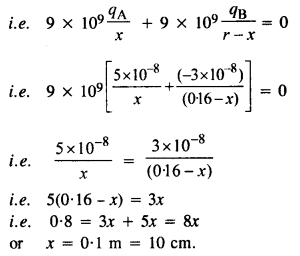Question 2.
A regular hexagon of side 10 cm has a charge 5 μC at each of its vertices. Calculate the potential at the center of the hexagon.
Total potential at O is given by,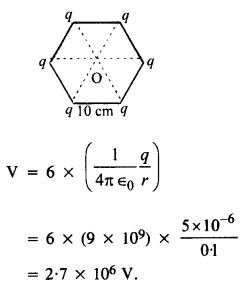Question 3.
Two charges 2μC and -2μC are placed at points A and B, 6 cm apart.
(a) Identify an equipotential surface of the system. % What is the direction of the electric field at every through the mid-point. On this plane, the potential is zero everywhere.
(b) The direction of the electric field is from positive to negative charge i.e. A to B, which is in fact perpendicular to the equipotential plane.
(a) A surface containing an equatorial line and a perpendicular line.
(b) Towards the side of – ve charge, parallel to the axis.

Question 4.
A spherical conductor of a radius of 12 cm has a charge of 1.6 x 10-7 C distributed uniformly on its surface. What is the electric field
(a) inside the sphere
(b) just outside the sphere
(c) at a point 18 cm from the center of the sphere?
(a) inside a conductor, the electric field is zero because the charge resides on the surface of a conductor.
(b) Electric field just outside the sphere is given by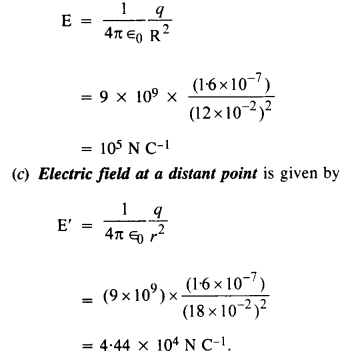Question 5.
A parallel plate capacitor with air between the plates has a capacitance of 8 pF
(1 pF = 10-12 F.) What will be the capacitance if the distance between the plates is reduced by half, and the space between them is filled with a substance of dielectric constant 6?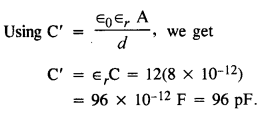Question 6.
Three capacitors each of capacitance 9 pF are connected in series.
(a) What is the total capacitance of the combination?
(b) What is the potential difference across each capacitor if the combination is connected to a 120 V supply ?Question 7.
Three capacitors of capacitances 2 pF, 3 pF and 4pF are connected in parallel.
(a) What is the total capacitance of the combination?
(b) Determine the charge on each capacitor if the combination is connected to a 100 V supply.
(a) Total capacitance
= c1 + c2 + c3
= 2 + 3+ 4 = 9pF.
(b) Using C = $$\frac { q }{ v }$$ we get q = CV
∴ qx = C1V = 2 x 10-12 x 100
= 2 x 10-10 C = 200 pC
q2 = c2V
= 3 x 10-12 x 100
= 3 x 10-10 C = 300 pC
q3 = c3v
= 4 x 10-12 x 100
= 4 x 10-10 C = 400 pC

Question 8.
In a parallel plate capacitor with air between the plates, each plate has an area of
6 x 10-3 in2 and the distance between the plates is 3 mm. Calculate the capacitance of the capacitor. If this capacitor is connected to a 100 V supply, what is the charge on each plate of the capacitor ?Question 9.
Explain what would happen if in the capacitor given in Q. 2.8, a 3 mm thick mica sheet (of dielectric constant = 6) were inserted between the plates,
(a) while the voltage supply remained connected.
(b) after the supply was disconnected.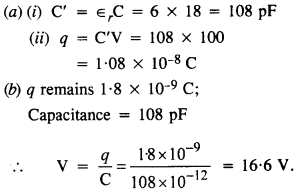Question 10.
A 12 pF capacitor is connected to a 50 V battery.How much electrostatic energy is stored in the capacitor ?
E = $$\frac { 1 }{ 2 }$$ CV2 = $$\frac { 1 }{ 2 }$$ x 12 x 10-12 x 50 x 50 = 1.5 2 2 x 10-8J.

Question 11.
A 600 pF capacitor is charged by a 200 V supply. It is then disconnected from die supply and is connected  to another uncharged 600 pF capacitor. How much electrostatic energy is lost in the process ?
Ans.
Here, C1 = 600pF = 6 x 10-10 F, C2 =
6 x 10-10 F, V1 = 200V, V2 = 0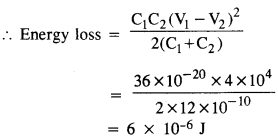Question 12.
A charge of 8 mC is located at the origin. Calculate the work done in taking a small charge of -2 x 10-9 C from a point P(0, 0, 3 cm) to a point Q (0, 4 cm, 0), via a point
R(0, 6 cm, 9 cm.)
The work done by electrostatic force on a charge is independent of the path followed by the charge. It depends only on the initial and final positions of the charge.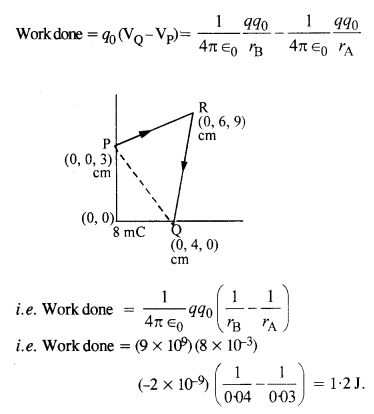Question 13.
A cube of side b has a charge q at each of its vertices. Determine the potential and electric field due to this charge array at the center of the cube.
(1) Distance of the center of the cube from vertex is half of the diagonal of the cube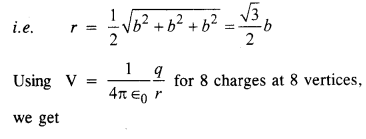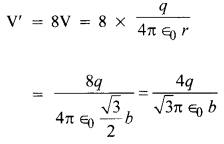(2) From symmetry, it is clear that electric field at center of the cube is zero.

Question 14.
Two tiny spheres carrying charges 1.5 μc and 2.5 μc are located 30 cm apart. Find the potential and electric field :
(a) at the mid point of the line joining the two charges,
(b) at a point 10 cm from this mid-point in a plane normal to the line and passing through the mid­point.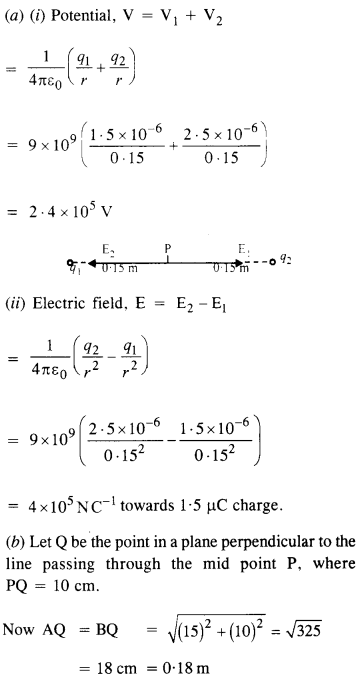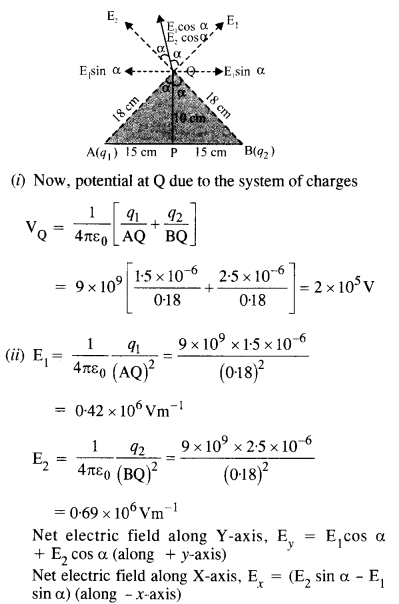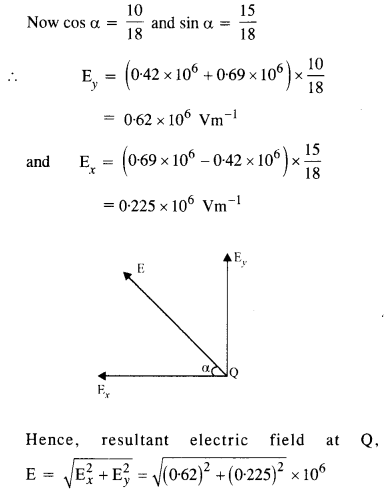Question 15.
A spherical conducting shell of inner radius rl and outer radius r2 has a charge Q.
(a) A charge q is placed at the center of the shell. What is the surface charge density on the inner and outer surfaces of the shell?
(b) Is the electric field inside a cavity (with no charge) zero, even if the shell is not spherical, but has any irregular shape? Explain.
(a) Charge Q appears on the outer surface.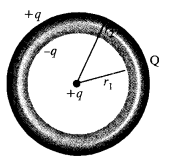When charge q is placed at the center, it induces – q charge on the inner surface and +q on the outer surface.
.’. charge density of the inner surface,and charge density of the outer surface,Consider a cavity of an irregular shape with the net charge to be zero inside it. Let a closed-loop be partially inside and the rest outside the cavity. The field inside the conductor is zero, so some work is done by the field to carry a test charge in the closed-loop, but this is against the provisions of an electrostatic field because as per Gauss’s law, the net charge inside a Gaussian surface must be zero. Thus, there cannot be field lines inside the cavity irrespective of its shape.

Question 16.
(a) Show that the normal component of the electrostatic field has a discontinuity from one side of a charged surface to another given by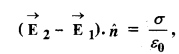where $$\hat { n }$$ is a unit vector normal to the surface at a point and a is the surface charge density at that point. (The direction of h is from side 1 to side 2.) Hence show that just outside a conductor, the electric field is σ$$\hat { n }$$ /ε0
(b) Show that the tangential component of the electrostatic field is continuous from one side of a charged surface to another.[Hint. For (a), use Gauss’s law. For, (b) use the fact that work done by electrostatic field on a closed loop is zero.]
Consider a sheet of charge having charge density a. E on either side of the sheet, perpendicular to the plane of sheet, has same magnitude at all points equidistant from the sheet.
Electric field intensity on the left side of the sheet,The electric field tangential to the plate is continuous throughout.

Question 17.
a long charged cylinder of linear charged density A. is surrounded by a hollow co-axial conducting cylinder. What is the electric field in the space between the two cylinders?
A cylinder P has linear charge density, λ, length l, and radius r1
The charge on cylinder P, q = XL A hollow co-axial conducting cylinder of length / and radius r2 surrounds the cylinder P. Charge on cylinder Q = – q.Consider a Gaussian surface in the form of a cylinder of radius r and length l. The electric flux through the curved surface of the Gaussian surface,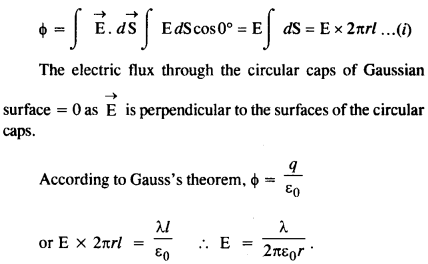Question 18.

In a hydrogen atom, the electron and proton are bound at a distance of about 0.53 Å:
(a) Estimate the potential Energy of the system in eV, taking the zero of the potential energy at an infinite separation of the electron from the proton.
(b) What is the minimum work required to free the electron, given that it’s kinetic energy in the orbit is half the magnitude of potential energy obtained in (a)?
(c) What are the answers to (a) and (b) above if the zero of potential energy is taken at 1.06 A separation?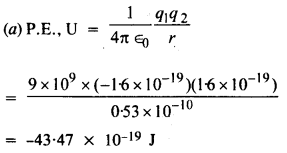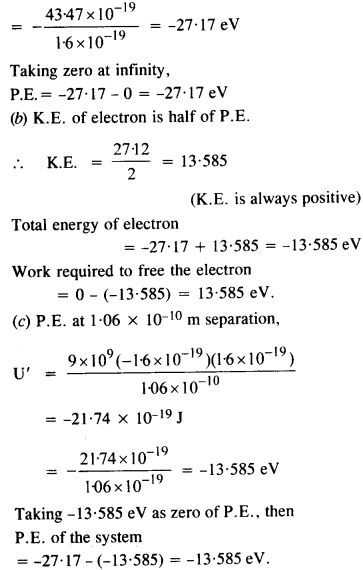Question 19.
If one of the two electrons of an H2 molecule is removed, we get a hydrogen molecular ion H+2. In the ground state of an H+2, the two protons are separated by roughly 1.5 Å, and the electron is roughly Å from each proton. Determine the potential energy of the system. Specify your choice of zero potential energy.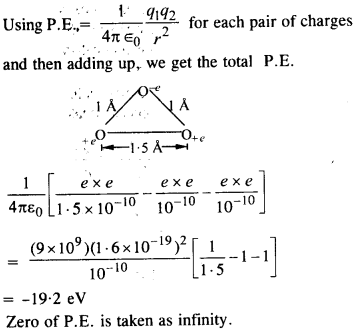Question 20.
Two charged conducting spheres of radii a and b are connected to each other by a wire. What is the ratio of the electric field at the surfaces of the two spheres? Use the result obtained to explain why the charge density on the sharp and pointed ends of a conductor is higher than on its flatter portions.
Two charged conducting spheres of radii a and b connected by a wire will reach to the same potential.Clearly, electric charge density for the pointed surface will be more because a flat surface can be equated to a spherical surface of large radius and a pointed portion to a spherical surface of small radius.

Question 21.
Two charges -q and + q are located at points (0, 0, -a) and (0, 0, a), respectively.
(a) What is the electrostatic potential at the points (0, 0, z) and (x, y, 0)?
(b) Obtain the dependence of potential on the distance r of a point from the origin when r/a > > 1.
(c) How much work is done in moving a small test charge from the point (5, 0, 0) to (-7, 0, 0) along the x-axis? Does the answer change if the path of the test charge between the same points is not along the x-axis?The point (x, y, 0) is perpendicular to Z-axis, there­fore, the potential at (x, y, 0) is zero.
(b) Consider P to be the point of observation at a distance r from the center (O) of the electric dipole.
Let OP make an angle 0 with the dipole moment $$\overrightarrow { p }$$
and r1, r2 be the distances of point P from – q charge and + q charge respectively. Potential at P due to – q charge,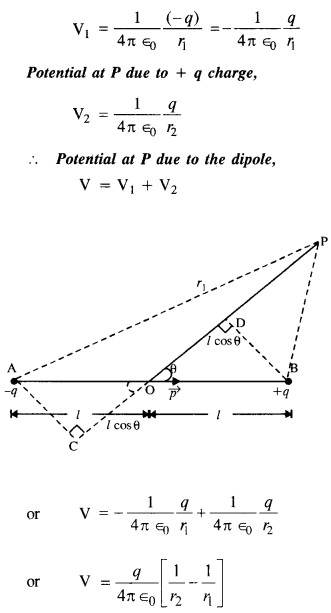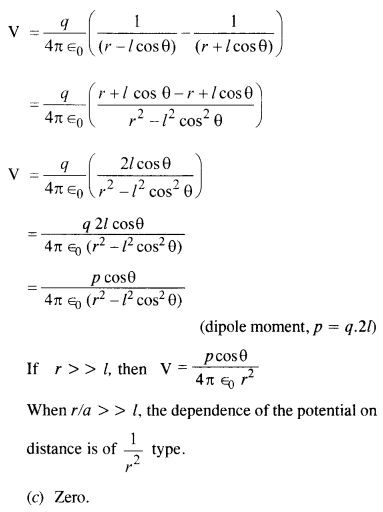The answer does not change because, in electrostatics, the work done does not depend upon the actual path, it simply depends upon the initial and final positions.

Question 22.
The figure shows a charge array known as an electric quadrupole. For a point on the axis of the quadrupole, obtain the dependence of potential on r for r/a > > l, and contrast your results with that due to an electric dipole, and an electric monopole
(i.e., a single charge.)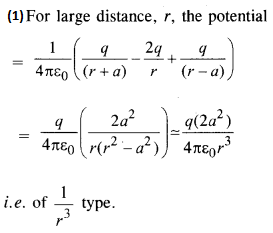(2) Due to electric dipole, the potential is of 1/r2 type.
(3) Due to an electric monopole, the potential is of 1/r type.

Question 23.
An electrical technician requires a capacitance of 2 μF in a circuit across a potential difference of 1 kV. A large number of 1 μF capacitors are available to him each of which can withstand a potential difference of not more than 400 V. Suggest a possible arrangement that requires the minimum number of capacitors.
Let N capacitors be used in m rows when each row has n capacitor i.e. N = mn
In series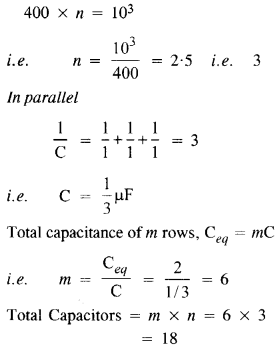Question 24.
What is the area of the plates of a 2 F parallel plate capacitor, given that the separation between the plates is 0.5 cm ? (You will realise from your answer why ordinary capacitors are in the range of μF or less. However, electrolytic capacitors do have a much larger capacitance ((0.1 F) because of very minute separation between the conductors.)Question 25.
Obtain the equivalent capacitance of the network in figure. For a 300 V supply, determine the charge and voltage across each capacitor. (C.B.S.E. 2008)The equivalent circuit is as shown below :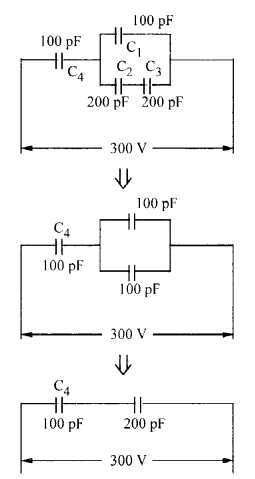Potential difference across C4 is in the ratio 2:1
i.e., 200 V
.’. Charge on C4 = C4V4
= 100 x 200 x 10-12 = 2 x 10-8 C
Potential difference across C1= 100 V
Charge on C1 = C1x V1
=100 x 100 x 10-12 = 1 x 10-8 C
Potential difference across C2 and C3 is 50 v each
∴ Charge on C2 or C3 = C2V2
= 200 x 50 x 10-12 = 10-8 C.

Question 26.
The plates of a parallel plate capacitor have an area of 90 cm2 each and are separated by 2.5 mm. The capacitor is charged by connecting it to a 400 V supply.
(a) How much electrostatic energy is stored by the capacitor?
(b) View this energy as stored in the electrostatic field between the plates, and obtain the energy per unit volume Hence arrive at a relation between u and the magnitude of electric field E between the plates.Question 27.
A 4 μF capacitor is charged by a 200 V supply. It is then disconnected from the supply and is connected to another uncharged 2 μF capacitor. How much electrostatic energy of the first capacitor is lost in the form of heat and electromagnetic radiation?Question 28.
Show that the force on each plate of a parallel plate capacitor has a magnitude equal to (1/2) QE, where Q is the charge on the capacitor and E is the magnitude of the electric field between the plates. Explain the origin of the factor (1/2).
Let F be the force on each plate of the capacitor. If the distance between the plates of the capacitor is increased by dx, then work done = F dx. This work done is stored as the potential energy of the capacitor. The increase in the volume of capacitor = A dxQuestion 29.
A spherical capacitor consists of two concentric spherical conductors held in position by suitable insulating supports (Figure.) Show that the capacitance of a spherical capacitor is given bywhere rx and r2 are the radii of outer and inner spheres, respectively.
It consists of two concentric spherical shells A and B of radii a and b with charge +q and charge -q respectively. (Outer sphere is grounded)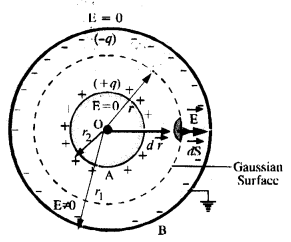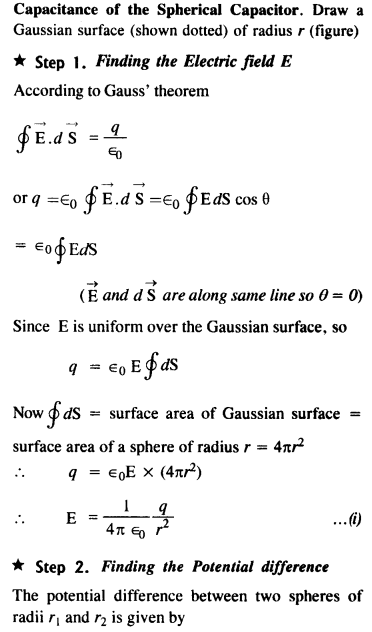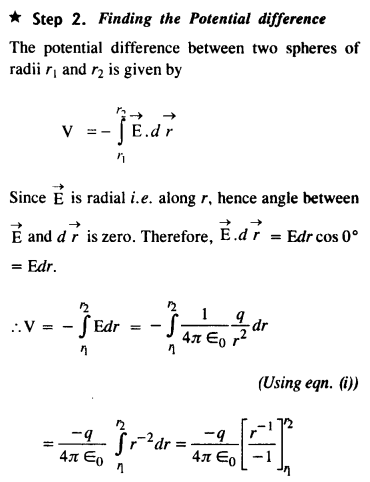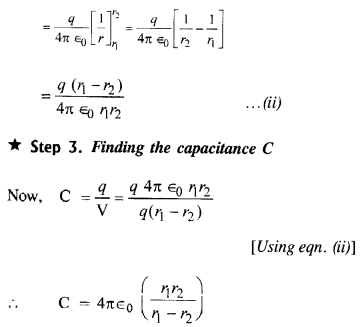Question 30.
A spherical capacitor has an inner sphere of radius 12 cm and an outer sphere of radius 13 cm. The outer sphere is earthed and the inner sphere is given a charge of 2.5 pC. The space between the concentric spheres is filled with a liquid of dielectric constant 32.
(a) Determine the capacitance of the capacitor.
(b) What is the potential of the inner sphere?
(c) Compare the capacitance of this capacitor with that of an isolated sphere of radius 12 cm. Explain why the latter is much smaller.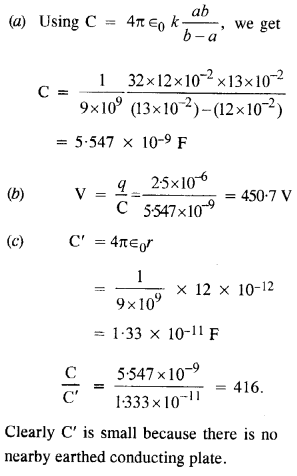Question 31.
(a) Two large conducting spheres carrying charges Q1 and Q2 are brought close to each other. Is the magnitude of the electrostatic force between them exactly given by Q1Q2/4Πε0r2, where r is the distance between their centers?
(b) If Coulomb’s law involved 1/r3 dependence (instead of 1/r2), would Gauss’s law be still true?
(c) A small test charge is released at rest at a point in an electrostatic field configuration. Will it travel along the field line passing through that point?
(d) What is the work done by the field of a nucleus in a complete circular orbit of the electron? What if the orbit is elliptical?
(e) We know that the electric field is discontinuous across the surface of a charged conductor. Is electric potential also discontinuous there?
(f) What meaning would you give to the capacitance of a single conductor?
(g) Guess a possible reason why water has a much greater dielectric constant (= 80) than say, mica (=6).
(a) No. The given relation is Coulomb’s law which is true for point charges. In the present case, as the spheres are brought closer, the distribution of charge on them becomes nonuniform.

(b) No. The surface area in space varies as r2 so that field varies as $$\frac { 1 }{ { r }^{ 2 } }$$. Hence $$\frac { 1 }{ { r }^{ 2 } }$$ dependence is essential.

(c) Not necessarily. The motion of charged particles need not be along the line of the field. It does so in the uniform field. The field gives the direction of acceleration and not that of velocity in general.

(d) Zero. For any complete path in the electrostatic field (the shape does not matter), it is zero.

(e) No. Potential is continuous there.

(f) The single conductor can form a condenser with the other conductor at infinity. Hence the meaning of storage of charge retains.

(g) Water molecules are polar molecules.

Question 32.
A cylindrical capacitor has two co-axial cylinders of length 15 cm and radii 1.5 cm and 1.4 cm. The outer cylinder is earthed and the inner cylinder is given a charge of 3.5 pC. Determine the capacitance of the system and the potential of the inner cylinder. Neglect end effects, (i.e., bending of field lines at the end)
The capacitance of a cylindrical capacitor is given by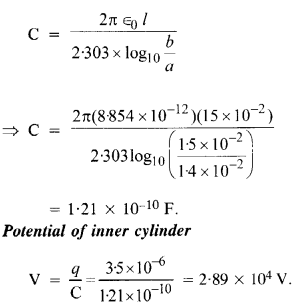Question 33.

A parallel plate capacitor is to be designed with a voltage rating of 1 kV, using a material of dielectric constant 3 and dielectric strength of about 107 Vm-1. (Dielectric strength is the maximum electric field a material can tolerate without breakdown, i.e., without starting to conduct electricity through partial ionization.) For safety, we should like the field never to exceed, say 10% of the dielectric strength. What minimum area of the plates is required to have a capacitance of 50 pF?
10% of the given field i.e. 107 V m1 gives E = 0.1 X 107 Vnr1Question 34.
Describe schematically the equipotential surfaces corresponding to
(a) a constant electric field in the z-direction,
(b) a field that uniformly increases in magnitude but remains in a constant (say, z) direction,
(c) a single positive charge at the origin, and
(d) a uniform grid consisting of long equally spaced parallel charged wires in a plane.
(a) A plane parallel to XY plane.
(b) Plane parallel to XY plane but the planes having different fixed potential will become closer with the increase in field intensity.
(c) Concentric spheres with origin as the center.
(d) A time-dependent changing shape nearer to the grid which slowly becomes planar and parallel to the grid at far off distances from the grid.

Question 35.
In a van de Graaff type generator a spherical metal shell is to be a 15 X 106 V electrode. The dielectric strength of the gas surrounding the electrode is 5 x 107 vm-1. What is the minimum radius of the spherical shell required? (You will learn from this exercise why one cannot build an electrostatic generator using a very small shell which requires a small charge to acquire a high potential.) (C.B.S.E. 2008)
The minimum radius of the shell of the van de Graaff generator is given by the relation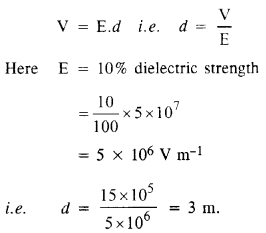Question 36.
A small sphere of radius r1 and charge q1 is enclosed by a spherical shell of radius r2 and charge q. Show that if q1 is positive, the charge will necessarily flow from the sphere to the shell (when the two are connected by a wire) no matter what the charge
q2 on the shell is.
Charge resides on the outer surface of a conductor. So the charge on the inner sphere will flow towards the shell through the conducting wire. Moreover, from Gauss’s law, no electric field exists inside a Gaussian surface, and also the charges enclosed by a closed surface only contribute towards the field. So q2 does not matter in this case. It is positive, a potential difference is also positive.

Question 37.
(a) The top of the atmosphere is at about 400 kV with respect to the surface of the earth, corresponding to an electric field that decrases with altitude. Near the surface of the earth, the field is about 100 Vm_1. Why then do we not get an electric shock as we step out of our house into the open ? (Assume the house to be a stell cage so there is no field inside !)
(b) A man fixes outside his house one evening a two meter high insulating slab carrying on its top a large aluminium sheet of area 1 m2. Will he get an electric shock if he touches the metal sheet next morning ?
(c) The discharging current in the atmosphere due to the small conductivity of air is known to be 1800 A on an average over the globe. Why then does the atmosphere not discharge itself completely in due course and become electrically neutral ? In other words, what keeps the atmosphere charged ?
(d) What are the forms of energy into which the electrical energy of the atmosphere is dissipated during a lightning ?
[Hint. The earth has an electric field of about 100 Vm-1 at its surface in the downward direction, corresponding to a surface charge density = -10-9 C m-2. Due to the slight conductivity of the atmosphere up to about 50 km (beyond which it is good conductor), about +1800 C is pumped every second into the earth as a whole. The earth, however, does not get discharged since thunderstorms and lightning occurring continually all over the globe pump an equal amount of negative charge on the earth.]